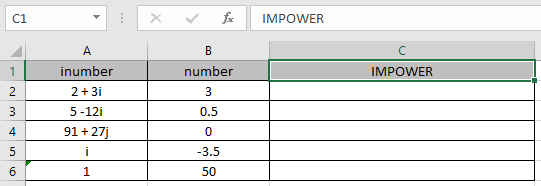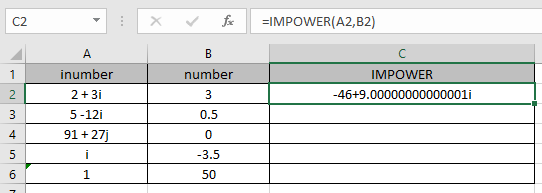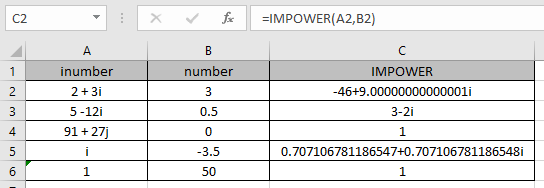# How to use the IMPOWER Function in Excel

In this article, we will learn about how to use the IMPOWER function in Excel.

COMPLEX number (inumber) in excel derived for mathematical number having real and imaginary coefficients. In mathematics we call it the coefficient of i or j (iota).

i = √-1

Square root of negative number is not possible, so for calculation purpose, √-1 is named as imaginary and call it iota (i or j). For calculation of some term like shown below.

= 2 +√-25

= 2 +√-1*25

= 2 +√-1*25

= 2 +√-1* 5

= 2 + 5i

This here equation is a Complex number (inumber) having 2 different parts called real part imaginary part

The coefficient of iota (i) which is 5 is called as imaginary part and the other part 2 is called the real part of the complex number.

Complex number (inumber) is written in the X iY format.

Power n of a complex number (X + iY) is given by

Power n of ( X iY ) = rn einΘ = rn Cos (nΘ) + i rn Sin(nΘ)

Here

1. X & Y are the coefficients of the real & imaginary part of the complex number (inumber) & n is the input number.
2. r is equal to the sum of the square of the real & imaginary part.

r = √X2 + Y2

1. Cos & Sin are the trigonometric characters where is equals to the inverse tangent of ( Y / X )

Θ = tan-1( Y / X)
The IMPOWER function returns the power ( number ) of the complex number (inumber) having both real & imaginary part.

Syntax:

=IMPOWER (inumber , number)

inumber : complex number having both real & imaginary
number : number representing the power n of a complex number
Let’s understand this function using it in an example.Here we have values where we need to get the power n of the input complex number (inumber)
Use the formula:

=IMPOWER ( A2 , B2 )

A2 : complex number ( inumber ) provided as cell reference.
B2 : complex number ( number ) provided as cell reference.As you can see the complex number having real_num = 2 & imaginary part = 3. The formula returns the complex Power 3 of the complex number.

Power 3 of ( 2 3i ) = r3ei3Θ= r3 Cos (3Θ) + i r3 Sin(3Θ)
Where r = √22 + 32= √13
&
Θ = tan-1( 3 / 2 )

Now copy the formula to the other remaining cells using Ctrl + D shortcut key.As you can see the IMPOWER function formula giving results just fine.

Use the Power 2 to get the Square of the complex number
& ½ to get the square root of the complex number.
USe the Power 3 to get the cube if the complex number & ? to get the cube root of the complex number.
The table show here explains more about the results

 inumber Real part (X) Imaginary part (Y) POWER ( n ) IMPOWER i = 0 + 1i 0 1 -3.5 0.707 + 0.707 i 1 = 1 + 0i 1 0 50 1

Note :

1. The formula returns the #NUM! error if the complex number doesn’t have lower case i or j (iota).
2. The formula returns the #VALUE! Error if the complex number doesn’t have correct complex number format.

Hope you understood how to use IMPOWER function and referring cell in Excel. Explore more articles on Excel mathematical functions here. Please feel free to state your query or feedback for the above article.

Related Articles
How to use the TYPE function
How to use the CELL function

Terms and Conditions of use

The applications/code on this site are distributed as is and without warranties or liability. In no event shall the owner of the copyrights, or the authors of the applications/code be liable for any loss of profit, any problems or any damage resulting from the use or evaluation of the applications/code.